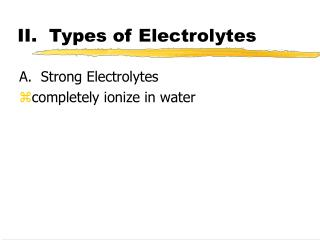DownloadDownload PresentationII. Types of Electrolytes

# II. Types of Electrolytes

Download Presentation## II. Types of Electrolytes

- - - - - - - - - - - - - - - - - - - - - - - - - - - E N D - - - - - - - - - - - - - - - - - - - - - - - - - - -
##### Presentation Transcript

1. II. Types of Electrolytes A. Strong Electrolytes • completely ionize in water

2. II. Types of Electrolytes A. Strong Electrolytes • completely ionize in water • conduct electricity well (bright light) AB + H2O  A+(aq) + B-(aq)

3. Types of Strong Electrolytes • Soluble salts • Strong acids: HCl, HI, HBr, HNO3, H2SO4, HClO4 HCl + H2O  H +(aq) + Cl-(aq) H2SO4 + H2O  H +(aq) + HSO4-(aq) • Strong bases: KOH, NaOH, Ba(OH)2, Ca(OH)2 KOH + H2O  K +(aq) + OH-(aq)

4. II. Types of Electrolytes B. Weak Electrolytes • Only some dissociation • Most remains intact in water • Don’t conduct electricity well (dim light)

5. Types of Weak Electrolytes • Weak acids: ex. HF, CH3CO2H and H3PO4 CH3CO2H CH3CO2-(aq) + H+(aq) • Weak bases: ex. Ammonia NH3 + H2O NH4+(aq) + OH-(aq)

6. II. Types of Electrolytes • C. Non- Electrolytes • dissolve in water but don’t form ions • do not conduct electricity (no light) • polar, non-ion forming compounds • ex. Sugar, ethanol

7. III. When Do Exchange Rx Occur? • A water insoluble product forms (a precipitation rx) • A stable molecule forms and removes ions from solution (ex. Neutralization rx-more details in chapt 11) • A gas is produced

8. IV. Precipitation Rx • A type of exchange reaction • soluble reactants form insoluble product

9. 3 Ways to Write Equations: • Overall eq AgNO3(aq) + KBr(aq) AgBr(s) + KNO3(aq) • Complete ionic Ag+ (aq) + NO3- (aq) + K+ (aq) + Br- (aq) AgBr(s) + K+ (aq) + NO3-(aq) • Net ionic Ag+ (aq) + Br-(aq) AgBr(s) cancel ions

10. How do you know when a solid forms? *Understand concepts and memorize rules! *Solids must have a net charge of zero-- 2 cations can’t form a solid.

11. Solubility Rules (memorize)

12. Predict the Solubility • KCl • MgCO3 • NiO • CaI2

13. Predict the Solubility • KCl soluble • MgCO3 insoluble • NiO insoluble • CaI2soluble

14. What Possible Solids Could Form in a Reaction? • Exchange anions and use solubility rules to see if products are solid or aqueous. • If both are aqueous there is no reaction.

15. Pb(NO3)2(aq) + K2CrO4(aq) ? For practice, write the overall, complete ionic and net ionic equations for this reaction.

16. Pb(NO3)2(aq) + K2CrO4(aq) ? Overall: Pb(NO3)2(aq) + K2CrO4(aq) PbCrO4(s) + 2KNO3(aq) Decide if any product is solid. Balance!!!

17. Pb(NO3)2(aq) + K2CrO4(aq) ? Overall: Pb(NO3)2(aq) + K2CrO4(aq) PbCrO4(s) + 2KNO3(aq) To do complete ionic, write aqueous species as separate ions. Complete ionic: Pb2+ (aq) + 2NO3- (aq) + 2K+ +CrO42-(aq) PbCrO4 (s) + 2K+ (aq) + 2NO3- (aq)

18. Pb(NO3)2(aq) + K2CrO4(aq) ? Overall: Pb(NO3)2(aq) + K2CrO4(aq) PbCrO4(s) + 2KNO3(aq) Complete ionic: Pb2+ (aq) + 2NO3- (aq) + 2K+ +CrO42-(aq) PbCrO4 (s) + 2K+ (aq) + 2NO3- (aq) Net ionic: Pb2+ (aq) + CrO42-(aq) PbCrO4 (s)